# Argentometry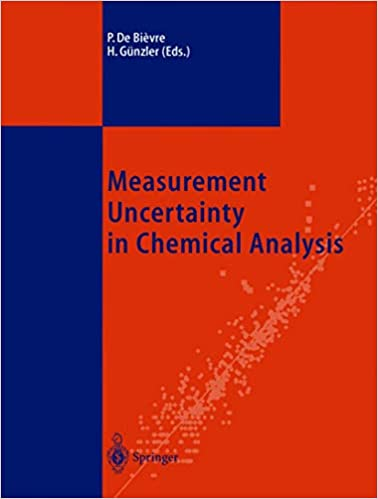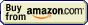## Precipitation titration » Curve calculation

According to the general guidelines we will calculate concentration before the equivalence point assuming titrant was a limiting reagent - thus concentration of titrated substance is that of unreacted excess. Usually that's already the answer, however, sometimes, instead of calculating concentration of titrated substance, we may want to calculate concentration of titrant. To do so it is enough to put concentration of excess titrated substance into solubility product and to solve for unknown concentration of titrant. After equivalence point situation reverses - if what we are looking for is a concentration of titrant, we simply calculate it from dilution of added titrant excess, if what we are looking for is a concentration of titrated substance - we put concentration of excess titrant into solubility product and we solve for unknown.

What is pAg in the 0.1 M AgNO3 solution titrated with 0.1 M HCl if 6 mL of titrant were added to the 20 mL sample.

According to the reaction equation

Ag+(aq) + Cl-(aq) → AgCl(s)

silver ions react with chloride 1:1. We started with 0.020L×0.1M = 0.002 moles (2 mmoles) of silver, and added to it 0.006L×0.1M = 0.0006 moles (0.6 mmole) of chlorides. Thus we are left with 2-0.6 = 1.4 mmole of silver in 24 mL of solution.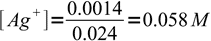1

and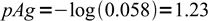2

What is pAg in the same titration when 21 mL of titrant were added. pKso = 9.8

Now we have 41 mL of total solution and 1 mL of excess titrant. That means 0.001L×0.1M = 0.0001 mole (0.1 mmole) of excess chlorides, or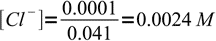3

Solubility product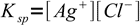4

solved for [Ag+]:5

and finally6

Page was last modified on February 25 2009, 15:47:09.

titration at www.titrations.info © 2009 ChemBuddy